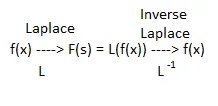# Mathematics - Laplace method for solving Differential equations

Hello it's a me again drifter1! Today we continue with Mathematics to talk about the Laplace method that let's us solve (mostly) any Differential equation! We will get into the method and theory behind it today, and next time into examples/exercises.

So, without further do, let's get straight into it!

## Laplace Transformation

Before getting into today's solving method we first need to talk about the Laplace tranformation in general!

For an function f: [0, +∞) -> R we define:This is the Laplace transformation of f(t) or f(x) if you want to use x.

The Laplace tranformation result is a function of s!

Supposing there is an inverse Laplace transformation we have:This means that we can get our function f(x) or f(t) back by using some inverse Laplace tranformation on the Laplace transformation result L(f(x)) or L(f(t)).

The most useful property of the transformation is that it is linear and so:

L[kf(x) +- λg(x)] = kL[f(x)] +- λL[g(x)]

Because the integral goes to infinity we calculate the Laplace transformation using:

L(f(x)) =Here (on wikipedia) you can read more about the Laplace transformation theorems and can also find a table/list of some transformations!

Transformations that you will need very often are:

• f(x) = c -> F(s) = c/s
• f(x) = x^n -> F(s) = n! / s^(n+1)
• f(x) = sin(ax) -> F(s) = a / (s^2 + a^2)
• f(x) = cos(ax) -> F(s) = s / (s^2 + a^2)
• f(x) = e^(ax) -> F(s) = 1 / (s-a)
• f(x) = e^(bx)*sin(ax) -> F(s) = a / [(s-b)^2 + a^2]
• f(x) = e^(bx)*cos(ax) -> F(s) = (s-b) / [(s-b)^2 + a^2]
• f(x) = x*sin(ax) -> F(s) = 2as / [s^2 + a^2]^2
• f(x) = x*cos(ax) -> F(s) = (s^2 - a^2) / [s^2 + a^2]^2
We also use these in the inverse Laplace transformation!

For example:

f(x) = e^(-2x) * sin5x => 5 / [(s+2)^2 + 25] = F(s)    [Laplace transformation]

F(s) = s / (s-1) => cos(-x) = f(x)    [Inverse Laplace transformation]

The Laplace transformation of an function f(x)=y is:

L(y) = Y

L(y') = s*Y - y(0)

L(y") = s^2*Y - s*y(0) - y'(0)

...

L(y^(n)) = s^n*Y - s^(n-1)*y(0) - s^(n-2)*y'(0) - ... - s* y^(n-2)(0) - y^(n-1)(0)

Which means that we need a starting condition y(0) and so y at x = 0.

## Solving differential equations

Suppose a constant coefficient linear 2nd-order ODE:

ay" + by" + cy = R(x)

Let's find the Laplace transformation for each side:

L(ay" + by" + cy) = L(R(x)) =>

aL(y") + bL(y') + cL(y) = L(R(x)) =>

... do some calculations

That way we end up with Y(s) = ...

Using the inverse Laplace transformation we can then find y(x).

These method can of course be applied in n-order Differential equations of any type and it doesn't have to be any of the cases we covered previously!

The difficulty comes in the calculations and in the way that we distinguish the different cases and transformations from each other.

So, to sum up the steps are:

1. Use the Laplace transformation on each side of the equation
2. Get to the form: Y(s) = ..., by solving for Y(s)
3. Use the inverse Laplace transformation to find y(x)

## Solving (differential) systems

Using the same method we can of course solve systems that contain derivatives now!

When having y(x) and z(x) be functions of x in a linear system of two equations (order 2) then:

1. we find the Laplace transformation for each equation independently.
2. we substitute each one in the other equation to end up with Y(s) = ... and Z(s) = ..., where each one doesn't contain the other.
3. we calculate the inverse Laplace transformation to get our y(x) and z(x) functions.

If the system is not so easy then we sometimes have to use some Linear algebra to solve the system of Laplace transformations (find Y and Z). I will get into a Linear algebra method of solving ODE's in general in a post later on!

Also, in my Systems and Signals series of Mathematics we will cover Laplace transformations more in depth and even more, but this will come much later on cause first I have to cover some more Mathematical Analysis and also Complex numbers!

Next time we will get into Exercises!

### Image sources:### Previous posts of the series:

Introduction -> Definition and Applications

First-order part(1) ->  Separable, homogeneous and exact 1st-order ODE's

First-order part(2) -> Linear, Bernoulli and Riccati first-order ODE's

First-order exercises -> Exercises for all the 1st-order ODE types

Second-order linear with const coeffs -> Constant coefficient linear 2nd-order ODE's

Special second-order forms -> Linear Euler ODE, Wronsky and Lagrange (Canonical) methods

Second-order exercises -> Exercises for 2nd-order ODE's

And this is actually it for today and I hope that you enjoyed it!

Next time we will apply this method in some exercises!

Bye!

H2
H3
H4
3 columns
2 columns
1 column
1 Comment